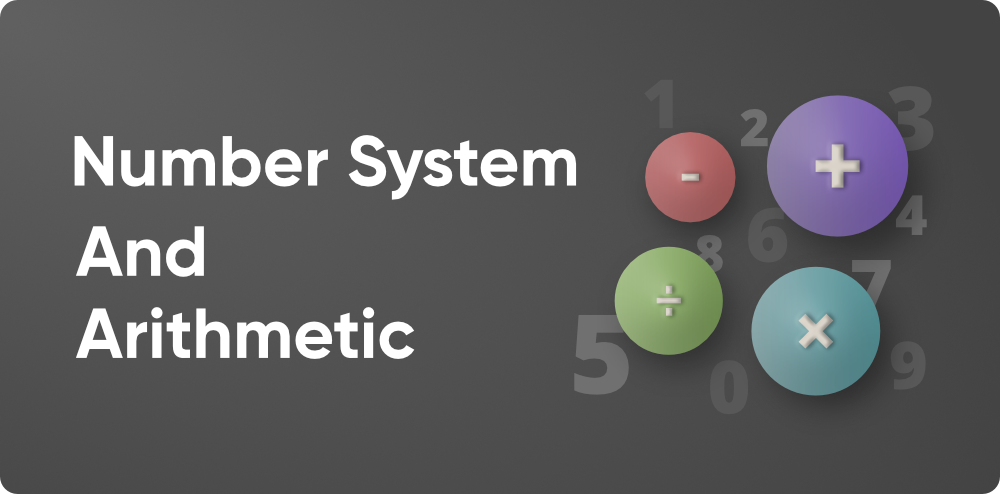Number System and Arithmetic

• Last Updated : 11 Nov, 2021

The number system is a system for representing numbers on the Number Line using a collection of symbols and rules. These symbols, which run from 0 to 9, are referred to as digits. The Number System is used to conduct mathematical computations ranging from complex scientific calculations to simple counting of Toys for a Kid or the number of chocolates left in the box.

However, Arithmetic is a field of mathematics that studies the characteristics of classical operations on numbers, such as addition, subtraction, multiplication, division, exponentiation, and root extraction. Arithmetic is a fundamental aspect of number theory, which, along with algebra, geometry, and analysis, is regarded as one of the premieres of modern mathematics. The phrases arithmetic and higher arithmetic were used as synonyms for number theory until the early twentieth century, and are now occasionally used to refer to a wider section of number theory.• Number System
• Arithmetic

Number System

Number System is a method of representing Numbers on the Number Line with the help of a set of Symbols and rules. These symbols range from 0-9 and are termed digits. Number System is used to perform mathematical computations ranging from great scientific calculations to calculations like counting the number of Toys for a Kid or Number chocolates remaining in the box. Number Systems comprise multiple types based on the base value for its digits. Further explanation about numbers and number system is provided below:

Arithmetic

Arithmetic is the foundation of how arithmetic works and how a person may use numbers to solve problems. It dives into the links between numbers and how they might affect one another when a person is trying to solve an issue. Learning more about arithmetic math, how it differs from other forms of math, the numerous topics a person will study, and how it can be used in the real world may help anybody decide why they want to learn more about it. It can also point them in the right direction if they need more support or want to learn something new. Anyone may extend their math skills to the moon and beyond by gradually studying more arithmetic. Following are the major topics covered in arithmetic along with their corresponding divisions:

Roots

Root, in mathematics, a solution to an equation, usually expressed as a number or an algebraic formula. There exists a special positive real number x such that xn = a, if a is a positive real number and n is a positive integer. So, n√a or a1/n is the number that represents the (principal) nth root of a. The integer n is referred to as the root’s index. When n = 2 is used, the root is referred to as the square root, and it is written as √a. The cube root of a is 3√a. The special negative nth root of an is called principal if an is negative and n is odd. The principal cube root of –27, for example, is –3. If a whole number (positive integer) has a logical nth root that is, one that can be expressed as a typical fraction, then the root must be an integer. Since 22 is less than 5, 5 has no rational square root. Here is a list of the topics that may help to understand roots more deeply:

Number Game

A number game is any of a variety of puzzles and games that include mathematical concepts. Mathematical games and puzzles range from simple amusements to complex problems, some of which have never been solved. Arithmetic, algebra, number theory, group theory, combinatory (problems of configurations or designs), symbolic logic, can all be included. The origins of number games and mathematical recreations, as well as the values on which they are based, are covered in details, such as explanations of the puzzles, games, and recreations are discussed in the articles below:

Ratio, Fractions, and Percentages

The discussions of ratio, proportion, and percentages in this free course are all about splitting everything into bits. For example, if there are 200 people in a small village and 50 of them are children, this can be expressed as a percentage: 25% of the village population is children; or as a ratio: one child for every four adults; or as a proportion: the proportion of children in the village population is a fifth. Following is the list of references that may help to understand more about this part of arithmetic:

Exponents

The term “exponents” refers to the repeated multiplication of the same element. The exponent, in this case, 3, represents the number of times the value is compounded. For instance, the shorthand for multiplying three copies of the number 2 is shown on the right-hand side of the equals sign as 2 × 2 × 2 = 8. The base is the thing that is being multiplied, which in this case is 5. Let’s learn more about the exponents in further articles as given below:

My Personal Notes arrow_drop_up